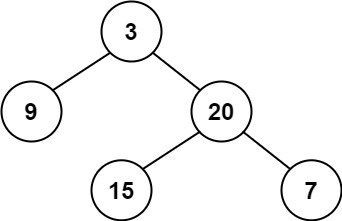Coding

# LeetCode Balanced Binary Tree Solution

0
(0)

Given a binary tree, determine if it is height-balanced.

For this problem, a height-balanced binary tree is defined as:

a binary tree in which the left and right subtrees of every node differ in height by no more than 1.

Example 1:```Input: root = [3,9,20,null,null,15,7]
Output: true
```

Example 2:```Input: root = [1,2,2,3,3,null,null,4,4]
Output: false
```

Example 3:

```Input: root = []
Output: true
```

Constraints:

• The number of nodes in the tree is in the range `[0, 5000]`.
• `-104 <= Node.val <= 104`

Solution

We need to find out if the binary tree is balanced or not. See carefully in the question that it is talking about binary tree and not binary search tree. Noting these kind of details in mind helps us avoid silly mistakes.

So what is a balanced binary tree. A balanced binary tree is a binary tree for which for any node, height of left subtree and right subtree will never differ by more than 1.

Now how did I solve this problem. Whenever I see a binary tree problem, the first thing that I use before anything else is recursion.

I find out if at the current node level (which root where we start), do the heights of left and right sub trees differ by 1 or less, and if the left subtree and right subtree are balanced, then my overall tree is balanced. Otherwise it is not.

Below is the Java code for above approach.

``````public boolean isBalanced(TreeNode root) {
if (root == null) return true;

return (Math.abs(height(root.left)-height(root.right)) <=1
&& isBalanced(root.left) && isBalanced(root.right));
}

private int height(TreeNode root) {
if (root == null) return 0;
return Math.max(height(root.left), height(root.right)) + 1;
}``````

And below is how fast it is compared to other LeetCode submitted solutions.

Click on a star to rate it!

Average rating 0 / 5. Vote count: 0

No votes so far! Be the first to rate this post.

As you found this post useful...

Follow us on social media!

We are sorry that this post was not useful for you!

Let us improve this post!

Tell us how we can improve this post?